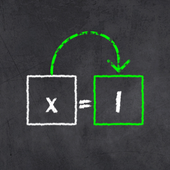# x=1: Learn to solve equations

0
|
0
Description of x=1: Learn to solve equations

For fun, to learn or to refresh your knowledge - with x=1 you can find the hard to catch X without any paperwork at all! All you have to do is to connect the elements of an equation step by step - of course only if the mathematical principles allow it. Additionally, you're free to use the equal sign as you like, so you can move things from one side of the equation to the other.

In x=1 you'll be able to solve equations build with the basic arithmetic operations + -, *, /. To spice things up, the rules of bracketing are also included. 40 exercises gradually teach you the mathematical principles - after that you'll be released into the wide world of equations to puzzle over an infinite number of randomly generated ones!
###### Category
Game » Educational
2.3.2
2020-08-08
Android 4.1+
v2.3.2 APK
4.14 MB
2020-08-08
v2.2 APK
3.80 MB
2020-08-03